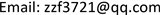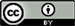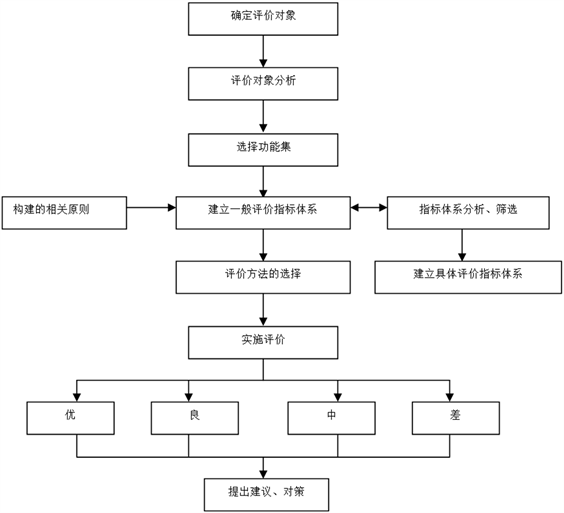﻿ 基于2011计划的高校体制机制协同创新绩效综合评价研究—以两型社会协同创新中心为例 A Comprehensive Evaluation of the Performance of University Institutional Mechanisms Based on the 2011 Plan—The Case study of Collaborative Innovation Center for Two-Oriented Society

Creative Education Studies
Vol.05 No.04(2017), Article ID:22530,11 pages
10.12677/CES.2017.54060

A Comprehensive Evaluation of the Performance of University Institutional Mechanisms Based on the 2011 Plan

—The Case study of Collaborative Innovation Center for Two-Oriented Society

Zhifang Zhou, Lei Nie, Yanfei Cai

Central South University Business School, Changsha HunanReceived: Oct. 9th, 2017; accepted: Oct. 23rd, 2017; published: Oct. 31st, 2017ABSTRACT

The reform of the university system in China has been one of the hot issues in the academic circles, and how to scientifically and reasonably evaluate the comprehensive performance of the University’s cooperative innovation is the key to measure the reform of the university system. Based on a lot of research and analysis, this paper constructs a comprehensive evaluation system of the system of university system, which is based on the “2011 plan”. The evaluation index system is divided into two parts: quantitative index and qualitative index system. The index weight is set up by using analytic hierarchy process. Finally, the empirical analysis is conducted with the example of the cooperative innovation center for two-oriented society.

Keywords:University, The 2011 Plan, The Reform of System and Mechanism, Performance Evaluation System

—以两型社会协同创新中心为例1. 引言

2. 综合评价流程与内容

2.1. 评价流程

2.2. 评价内容Figure 1. The performance evaluation process of the mechanism of collaborative innovation for university institutional based on the 2011 plan

3. 评价指标体系的构建

3.1. 构筑原则

1) 科学性与实用性相结合

2) 系统性与层次性相结合

3) 全面性与目的性相结合

4) 可比性与监控性相结合

3.2. 评价指标的选取Table 1. The performance evaluation index system of the mechanism of collaborative innovation for university institutional based on the 2011 planTable 2. The qualitative evaluation index of the mechanism of collaborative innovation for university institutional based on the 2011 plan

3.3. 指标体系权重的设置Table 3. The relative weight of quantitative evaluation index system of the mechanism of collaborative innovation for university based on the 2011 plan

ContinuedTable 4. The relative weight of qualitative evaluation index system of the mechanism of collaborative innovation for university based on the 2011 plan

3.4. 指标方法的选择

4. 实证研究——以两型社会协同创新中心为例

4.1. 中心简介

4.2. 综合绩效评价

Continued

$\text{A}=\text{A1}\ast 0.\text{14}+\text{A2}\ast 0.\text{43}+\text{A3}\ast 0.\text{43}=\text{1}.\text{779}$

$\text{B}=\text{B1}\ast 0.\text{11}+\text{B2}\ast 0.\text{48}+\text{B3}\ast 0.\text{41}=0.\text{369}$

$\text{C}=\text{C1}\ast 0.\text{19}+\text{C2}\ast 0.0\text{8}+\text{C3}\ast 0.\text{73}=0.\text{278}$

$\text{D}=\text{D1}\ast 0.\text{13}+\text{D2}\ast 0.\text{26}+\text{D3}\ast 0.\text{56}+\text{D4}\ast 0.0\text{5}=10.65$

$\begin{array}{l}\text{E}=\text{E1}\ast 0.0\text{5}+\text{E2}\ast 0.0\text{5}+\text{E3}\ast 0.0\text{5}+\text{E4}\ast 0.\text{13}+\text{E5}\ast 0.\text{15}+\text{E6}\ast 0.\text{15}\\ \text{\hspace{0.17em}}\text{\hspace{0.17em}}\text{\hspace{0.17em}}\text{\hspace{0.17em}}\text{\hspace{0.17em}}\text{\hspace{0.17em}}+\text{E7}\ast 0.\text{15}+\text{E8}\ast 0.\text{15}+\text{E9}\ast 0.0\text{6}+\text{E}10\ast 0.0\text{6}=1.09\end{array}$

$\text{F}=\text{F1}\ast 0.\text{63}+\text{F2}\ast 0.\text{13}+\text{F3}\ast 0.\text{13}+\text{F4}\ast 0.\text{13}=\text{1}.\text{26}$

$\text{M}=\text{A}\ast 0.0\text{8}+\text{B}\ast 0.0\text{5}+\text{C}\ast 0.0\text{4}+\text{D}\ast 0.\text{24}+\text{E}\ast 0.\text{46}+\text{F}\ast 0.\text{13}=\text{3}.\text{393}$

$\text{A ′}=\text{A1 ′}\ast 0.\text{14}+\text{A2 ′}\ast 0.\text{43}+\text{A3 ′}\ast 0.\text{43}=\text{3}.\text{3781}$

$\text{B ′}=\text{B1 ′}\ast 0.\text{11}+\text{B2 ′}\ast 0.\text{48}+\text{B3 ′}\ast 0.\text{41}=0.\text{7659}$

$\text{C ′}=\text{C1 ′}\ast 0.\text{19}+\text{C 2 ′}\ast 0.0\text{8}+\text{C 3 ′}\ast 0.\text{73}=0.\text{462}$

$\text{D ′}=\text{D1 ′}\ast 0.\text{13}+\text{D2 ′}\ast 0.\text{26}+\text{D3 ′}\ast 0.\text{56}+\text{D4 ′}\ast 0.0\text{5}=\text{16}.\text{93}$

$\begin{array}{l}\text{E ′}=\text{E1 ′}\ast 0.0\text{5}+\text{E2 ′}\ast 0.0\text{5}+\text{E3 ′}\ast 0.0\text{5}+\text{E4 ′}\ast 0.\text{13}+\text{E5 ′}\ast 0.\text{15}\\ \text{\hspace{0.17em}}\text{\hspace{0.17em}}\text{\hspace{0.17em}}\text{\hspace{0.17em}}\text{\hspace{0.17em}}\text{\hspace{0.17em}}\text{ }\text{ }+\text{E6 ′}\ast 0.\text{15}+\text{E7 ′}\ast 0.\text{15}+\text{E8 ′}\ast 0.\text{15}+\text{E9 ′}\ast 0.0\text{6}+\text{E}1{0}^{\prime }\ast 0.0\text{6}=\text{11}.\text{5}\end{array}$

$\text{F ′}=\text{F1 ′}\ast 0.\text{63}+\text{F2 ′}\ast 0.\text{13}+\text{F3 ′}\ast 0.\text{13}+\text{F4 ′}\ast 0.\text{13}=\text{7}.\text{76}$

$\text{M ′}=\text{A ′}\ast 0.0\text{8}+\text{B ′}\ast 0.0\text{5}+\text{C ′}\ast 0.0\text{4}+\text{D ′}\ast 0.\text{24}+\text{E ′}\ast 0.\text{46}+\text{F ′}\ast 0.\text{13}=10.689$

(1) 采用专家打分法获得定性指标评语集Table 6. The fuzzy comprehensive evaluation matrix before reformTable 7. The fuzzy comprehensive evaluation matrix after reform

5. 结论

2016年湖南省普通高等学校教学改革研究项目、2016年中南大学教育教学改革研究项目：高校“学生–学术–学科”三位一体综合评价体系构建研究——基于省2011计划视角(项目编号：2016jy53)。

A Comprehensive Evaluation of the Performance of University Institutional Mechanisms Based on the 2011 Plan—The Case study of Collaborative Innovation Center for Two-Oriented Society[J]. 创新教育研究, 2017, 05(04): 381-391. http://dx.doi.org/10.12677/CES.2017.54060

1. 1. Green, A. (1999) Education and Globalization in Europe an East Asia: Convergent and Divergent Trends. Journal of Education Policy, 14, 55-71. https://doi.org/10.1080/026809399286495

2. 2. Jacobs, B. (2006) How to Reform Higher Education in Europe. Economic Policy, 7, 539-592.

3. 3. Estermann, T. and Nokkala, T. (2009) University Autonomy in Europe: Exploratory Study. European University Association, Brussels, 26, 39-41.

4. 4. Birnbaum, R. (2004) The End of Shared Governance: Looking Ahead or Looking Back Matter. New Direction for Higher Education, 127, 12-43.

5. 5. Dobbins, M., Knill, C. and Vögtle, E.M. (2011) An Analytical Framework for the Cross-Country Comparison of Higher Education Governance. Higher Education, 62, 665-683. https://doi.org/10.1007/s10734-011-9412-4

6. 6. 丁烈云. 实施“2011”计划的意义、重点与难点[J]. 中国高等教育, 2012(20): 11-39.

7. 7. 王寰安. 我国高等教育体制改革为何成效不足[J]. 高等教育研究, 2011(4): 30-36.

8. 8. 马陆亭. 我国高等教育管理体制改革30年——历程、经验与思考[J]. 中国高教研究, 2008(11): 12-17.

9. 9. 毕宪顺, 赵凤娟, 甘金球. 教授委员会:学术权力主导的高校内部管理体制[J]. 教育研究, 2011(9): 45-50.

10. 10. 谌晓芹. 欧洲教育一体化改革: 博洛尼亚进程的结构与改革分析[J]. 高等教育研究, 2012(6): 92-100.

11. 11. 张园. 国家级协同创新中心新型科研组织模式发展[J]. 人民论坛, 2015(11): 85-87.

12. 12. 仲崇娜. 高校协同创新平台组织结构与运行机制研究[J]. 科技进步与对策, 2015(6): 29-34.

13. 13. Johnes, J. and Johnes, G. (1995) Research Funding and Performance in UK University Departments of Economics: A Frontier Analysis. Economics of Education Review, 14, 301-314. https://doi.org/10.1016/0272-7757(95)00008-8

14. 14. 戴航, 张培林, 孙孝文. 基于AHP-模糊综合评价法的物流园区选址研究[J]. 物流技术, 2014, 4(33): 98-100.

15. 15. 韩利, 梅强, 陆玉梅, 等. AHP-模糊综合评价方法的分析与研究[J]. 中国安全科学学报, 2004, 14(7): 86-89.

16. 16. 吴丽萍. 模糊综合评价方法及其应用研究[D]: [硕士学位论文]. 太原: 太原理工大学, 2006: 40-46.